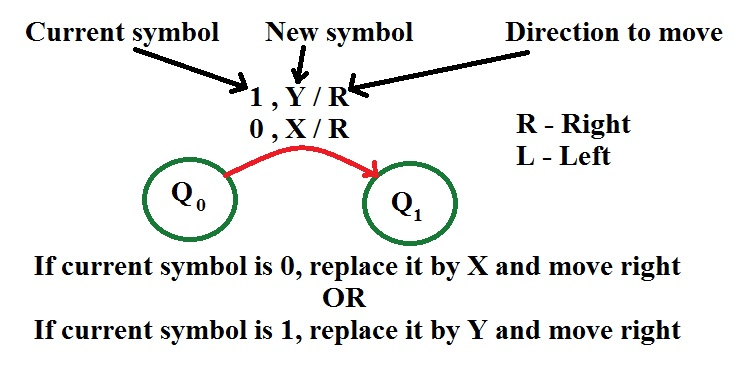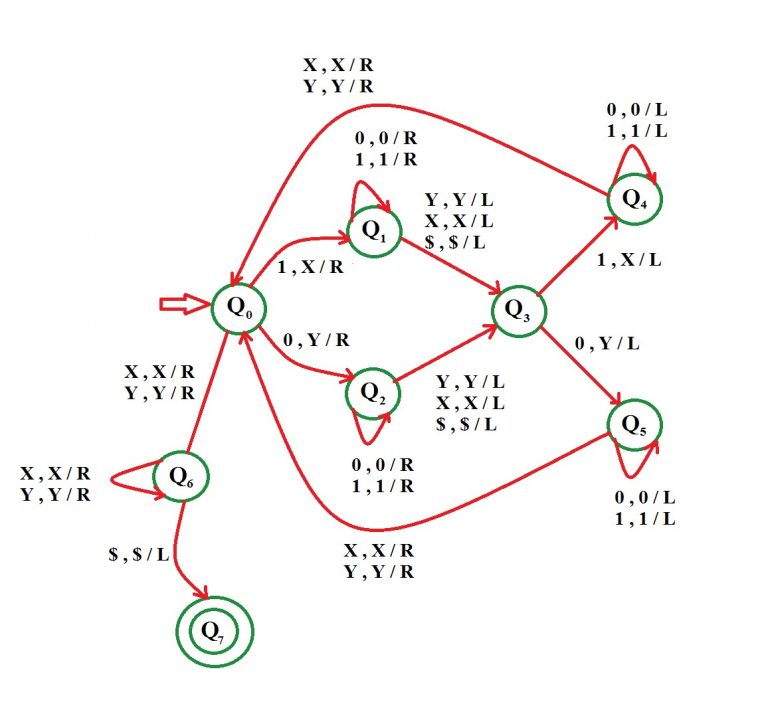# Construct a Turing Machine for language L = {wwr | w ∈ {0, 1}}

• Difficulty Level : Expert
• Last Updated : 08 May, 2018

Prerequisite – Turing Machine
The language L = {wwr | w ∈ {0, 1}} represents a kind of language where you use only 2 character, i.e., 0 and 1. The first part of language can be any string of 0 and 1. The second part is the reverse of the first part. Combining both these parts out string will be formed. Any such string which falls in this category will be accepted by this language. The beginning and end of string is marked by \$ sign.

For example, if first part w = 1 1 0 0 1 then second part wr = 1 0 0 1 1. It is clearly visible that wr is the reverse of w, so the string 1 1 0 0 1 1 0 0 1 1 is a part of given language.

Examples –

```Input : 0 0 1 1 1 1 0 0
Output : Accepted
Input : 1 0 1 0 0 1 0 1
Output : Accepted
```

Basic Representation –Assumption: We will replace 0 by Y and 1 by X.

Approach Used –
First check the first symbol, if it’s 0 then replace it by Y and by X if it’s 1. Then go to the end of string. So last symbol is same as first. We replace it also by X or Y depending on it.
Now again come back to the position next to the symbol replace from the starting and repeat the same process as told above.

One important thing to note is that since wr is reverse of w of both of them will have equal number of symbols. Every time replace a nth symbol from beginning of string, replace a corresponding nth symbol from the end.

• Step-1:
If symbol is 0 replace it by Y and move right, Go to state Q2
If symbol is 1 replace it by X and move right, Go to state Q1

• Step-2:
If symbol is 0 replace it by 0 and move right, remain on same state
If symbol is 1 replace it by 1 and move right, remain on same state
——————————————————————-
If symbol is X replace it by X and move right, Go to state Q3
If symbol is Y replace it by Y and move right, Go to state Q3
If symbol is \$ replace it by \$ and move right, Go to state Q3

• Step-3:
If symbol is 1 replace it by X and move left, Go to state Q4
If symbol is 0 replace it by Y and move left, Go to state Q5

• Step-4:
If symbol is 1 replace it by 1 and move left
If symbol is 0 replace it by 0 and move left
Remain on same state

• Step-5:
If symbol is X replace it by X and move right
If symbol is Y replace it by Y and move right
Go to state Q0

• Step-6:
If symbol is X replace it by X and move right
If symbol is Y replace it by Y and move right
Go to state Q6
ELSE
Go to step 1

• Step-7:
If symbol is X replace it by X and move right, Remain on same state
If symbol is Y replace it by Y and move right, Remain on same state
If symbol is \$ replace it by \$ and move left, STRING IS ACCEPTED, GO TO FINAL STATE Q7My Personal Notes arrow_drop_up